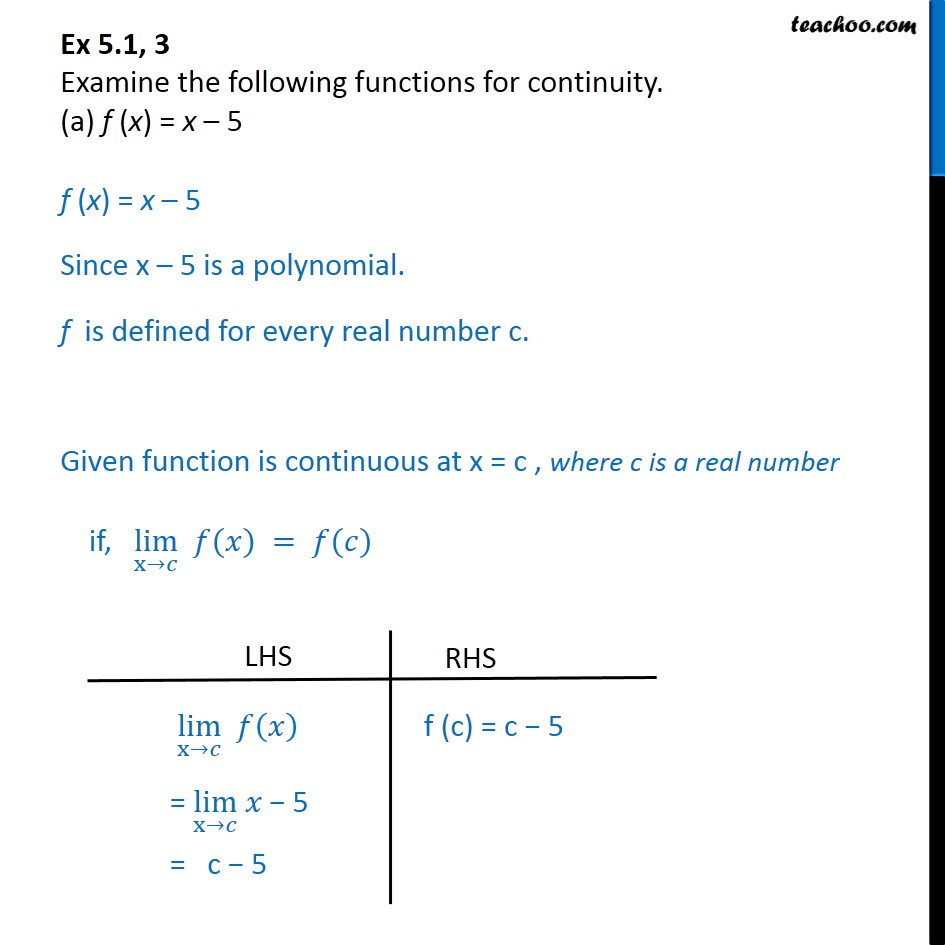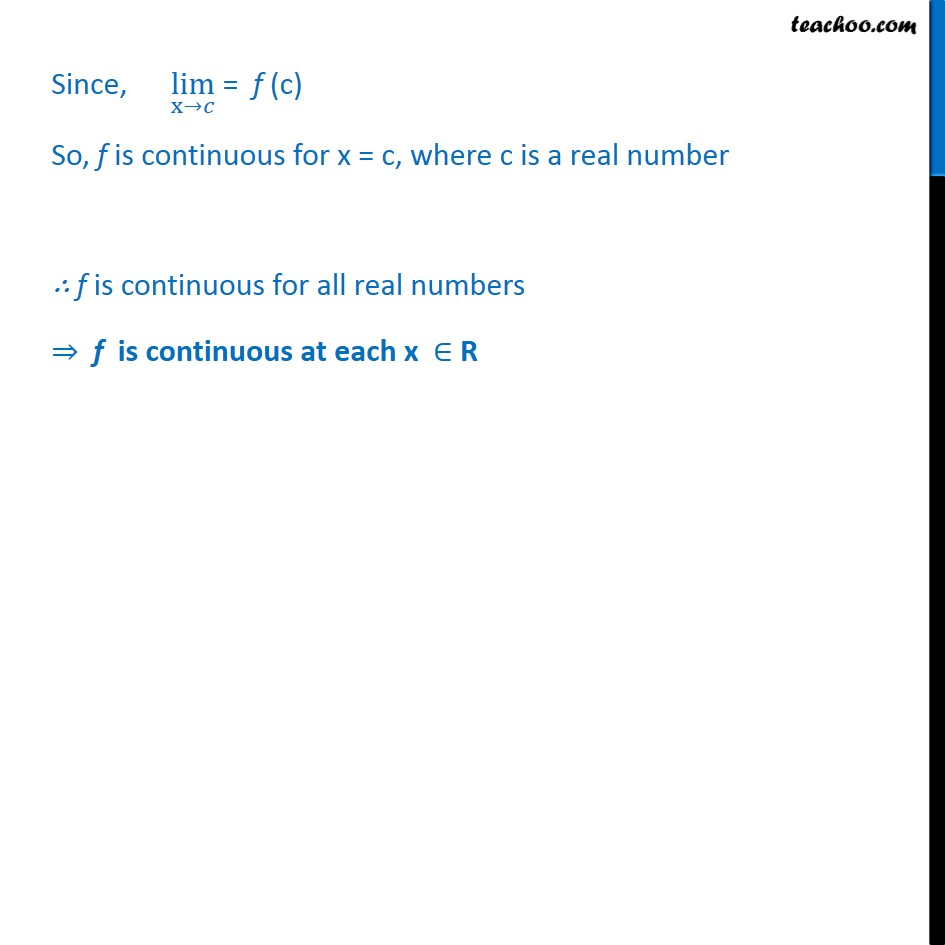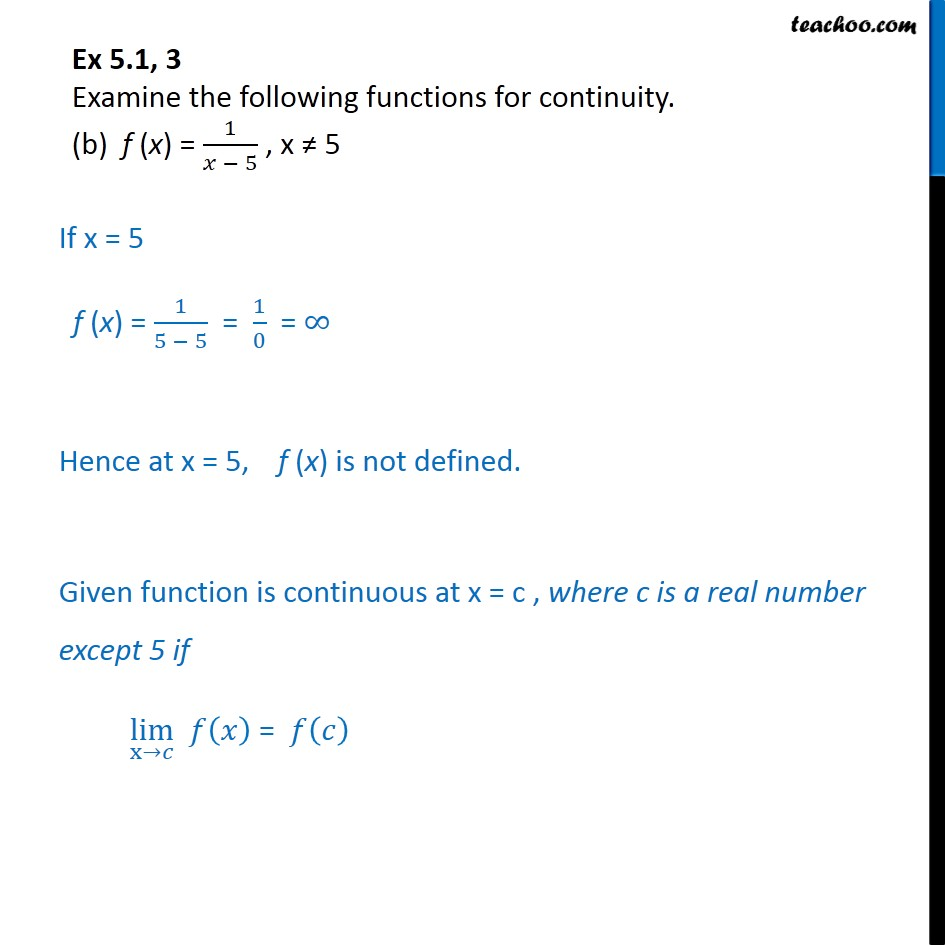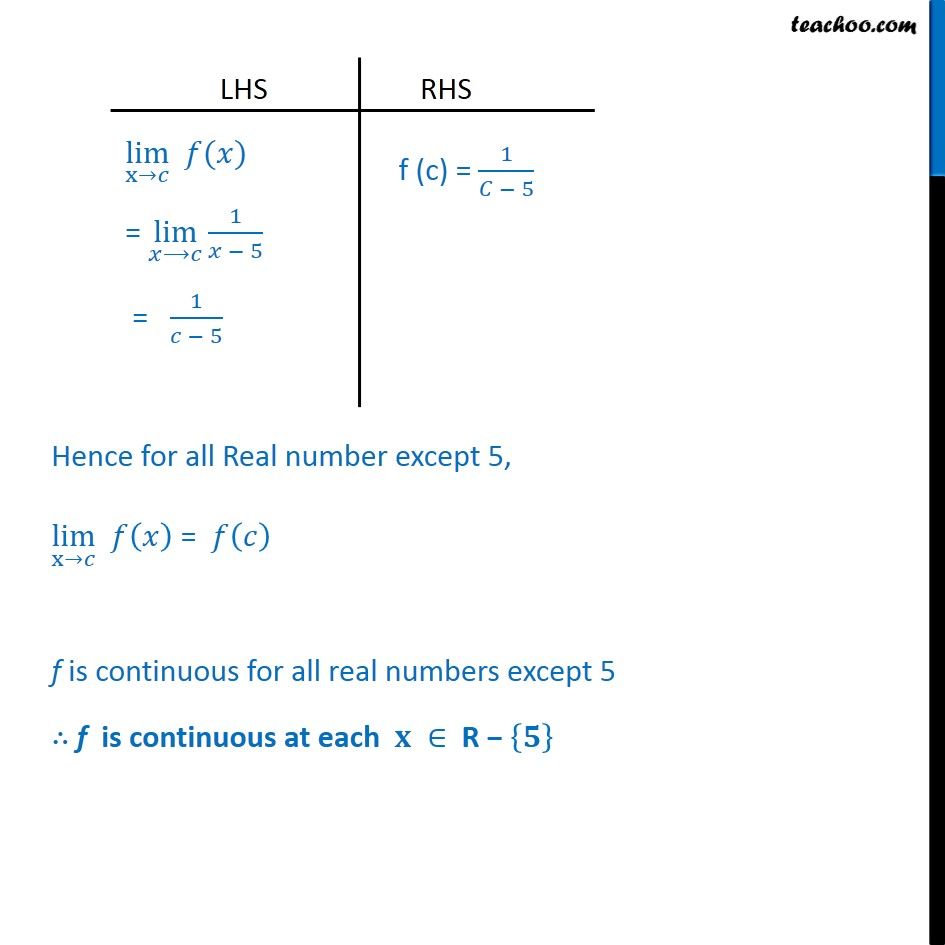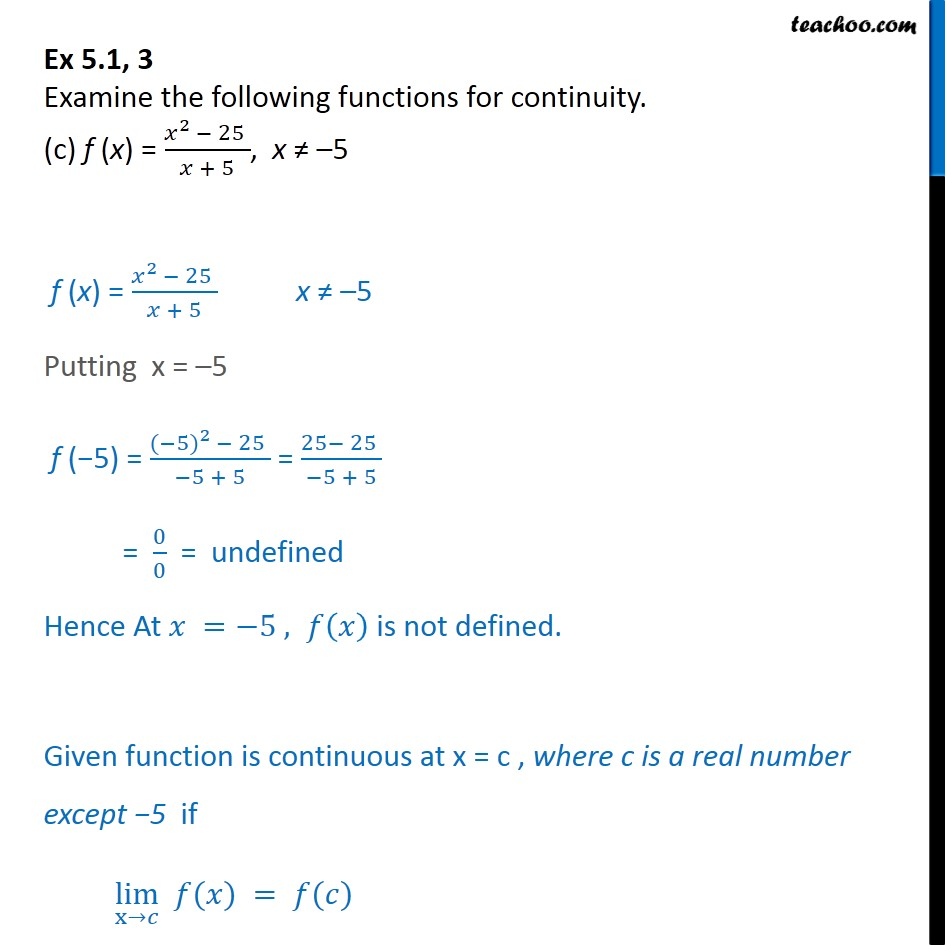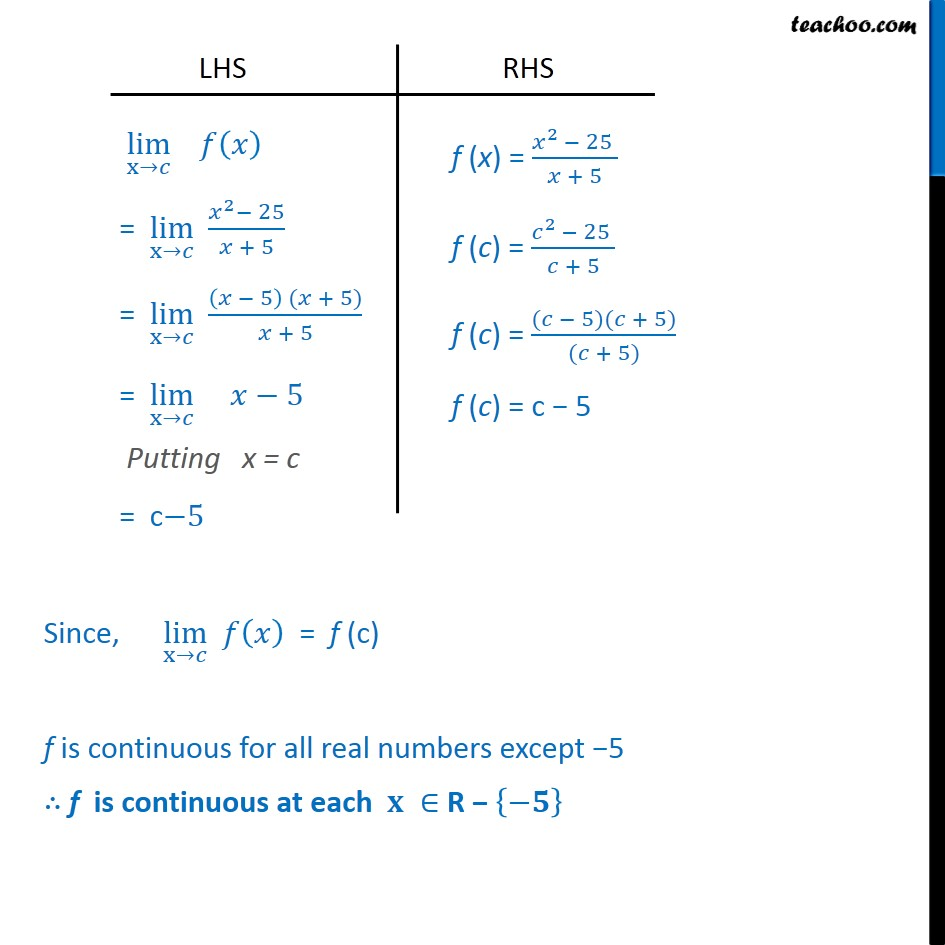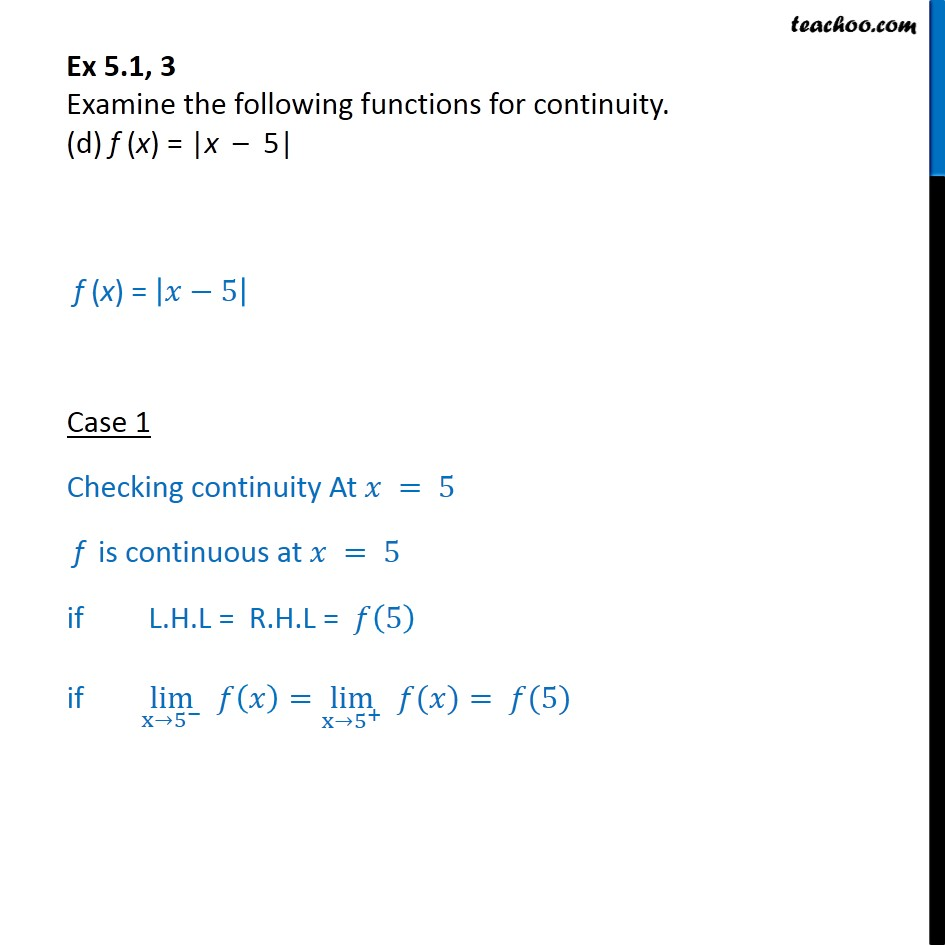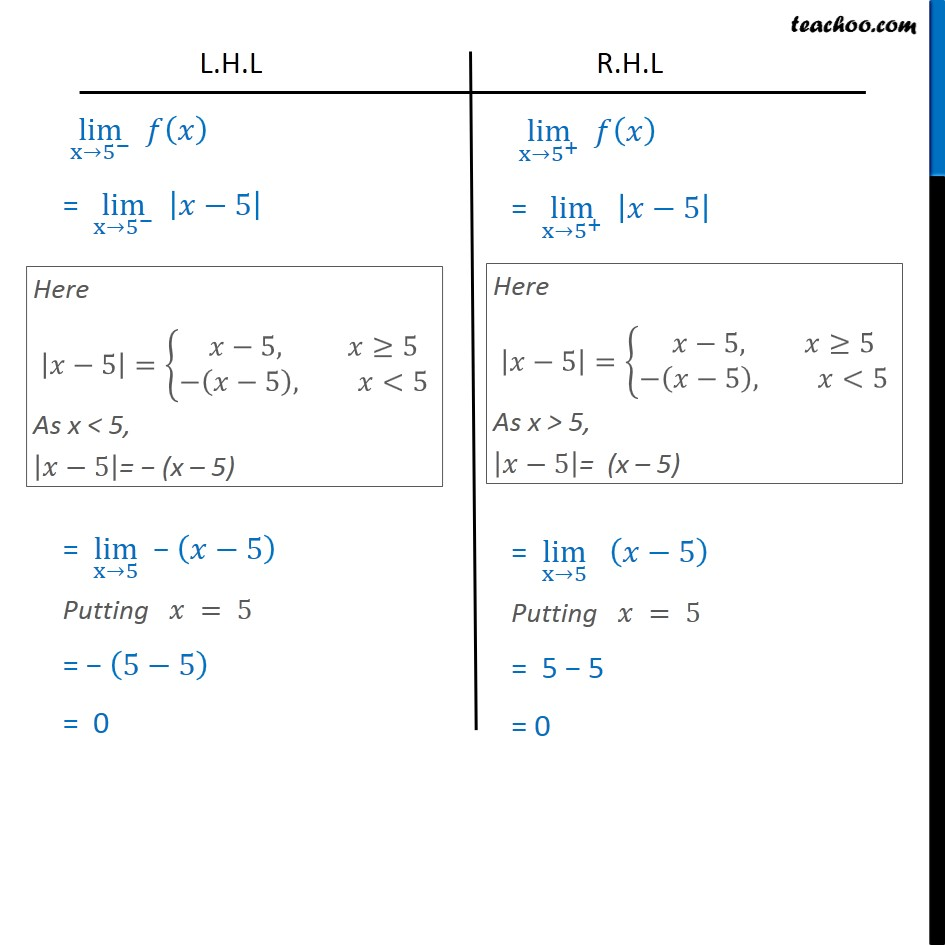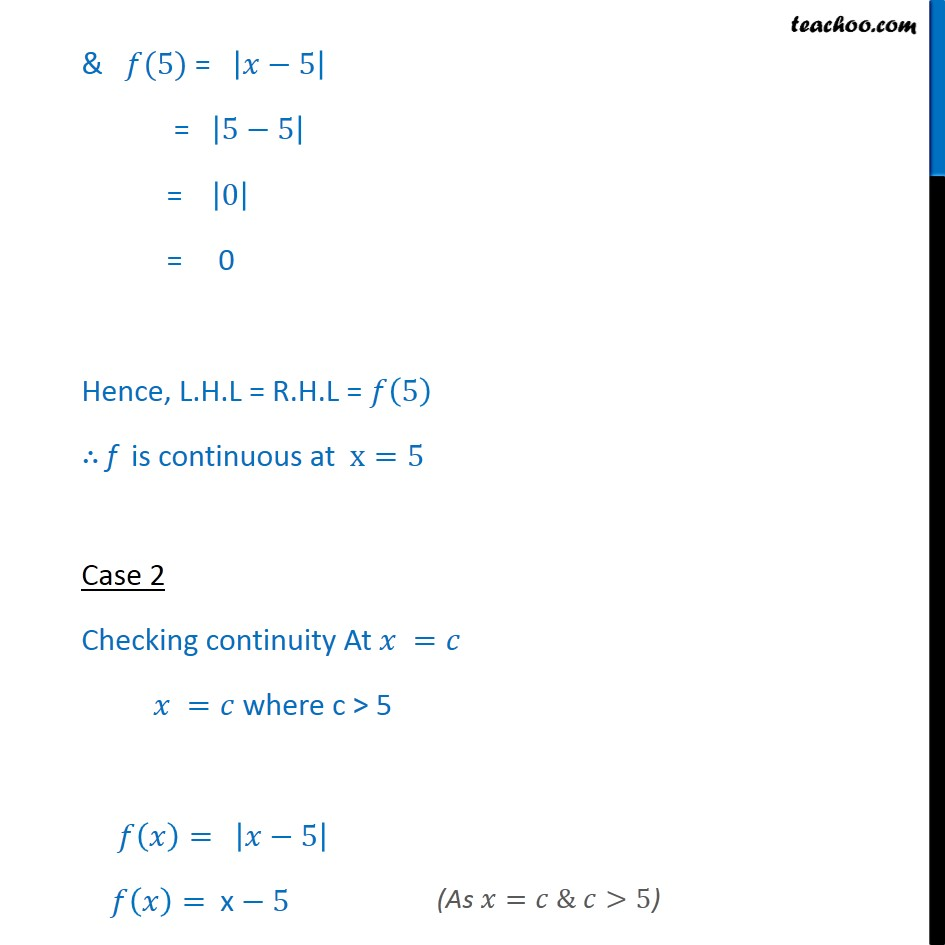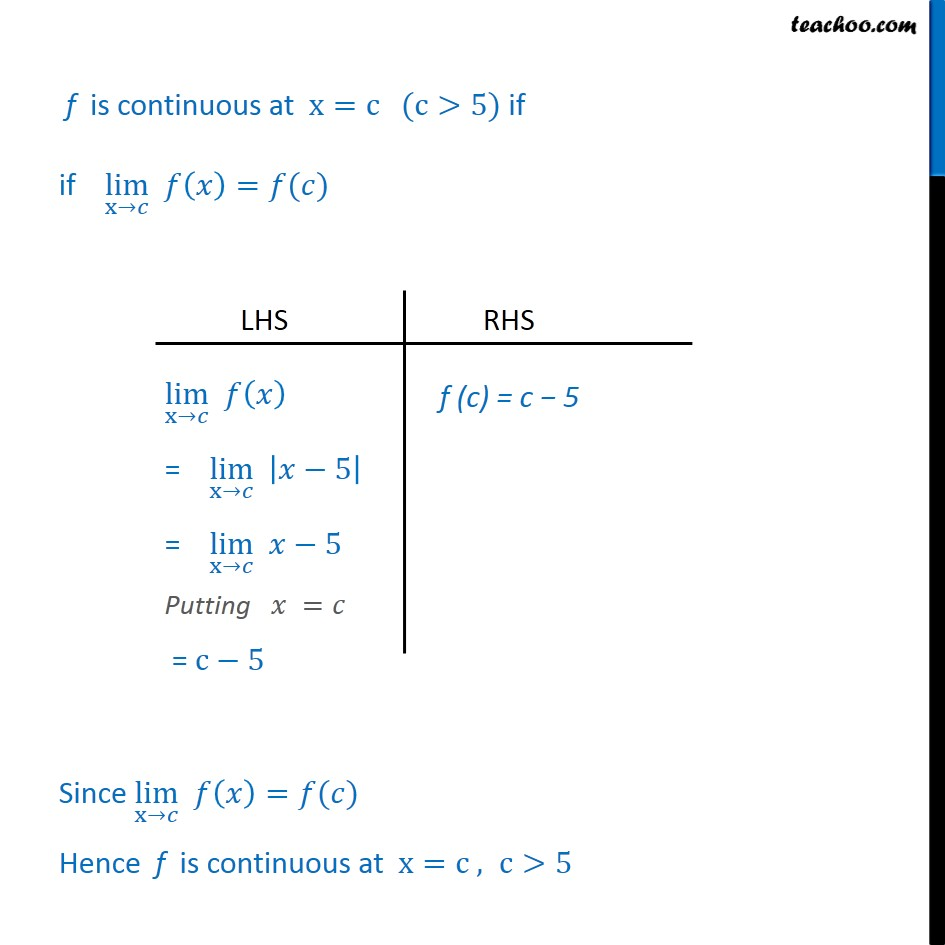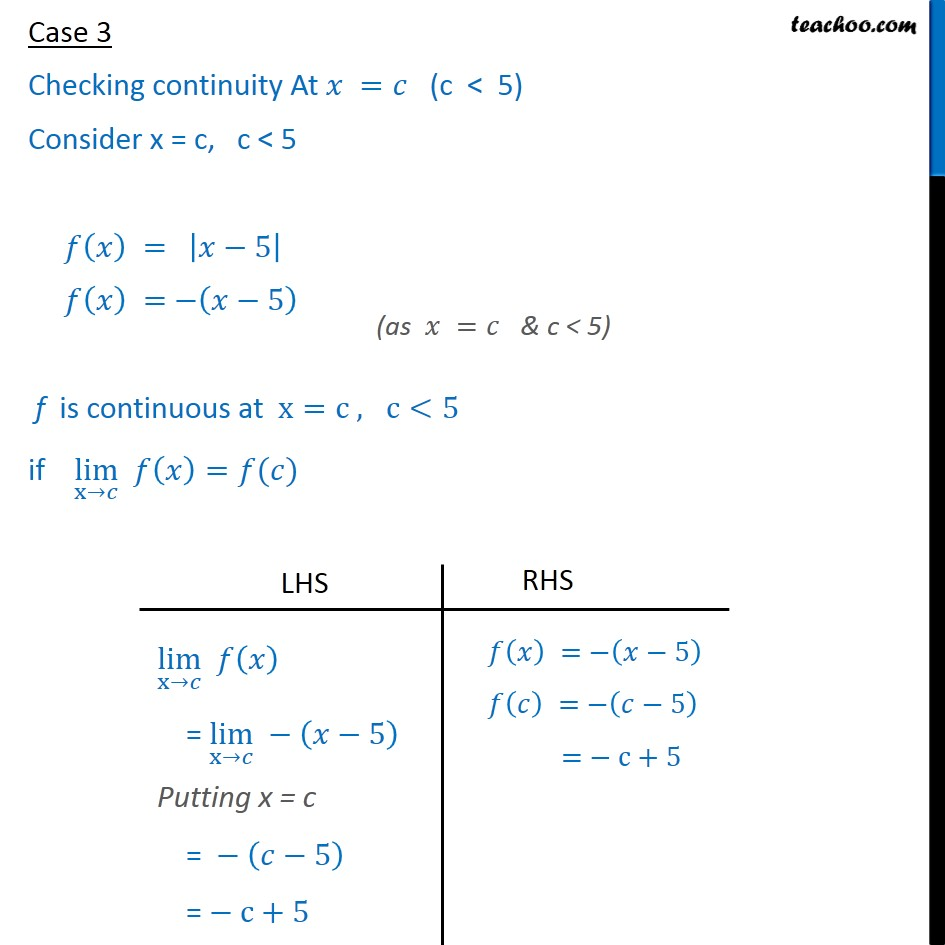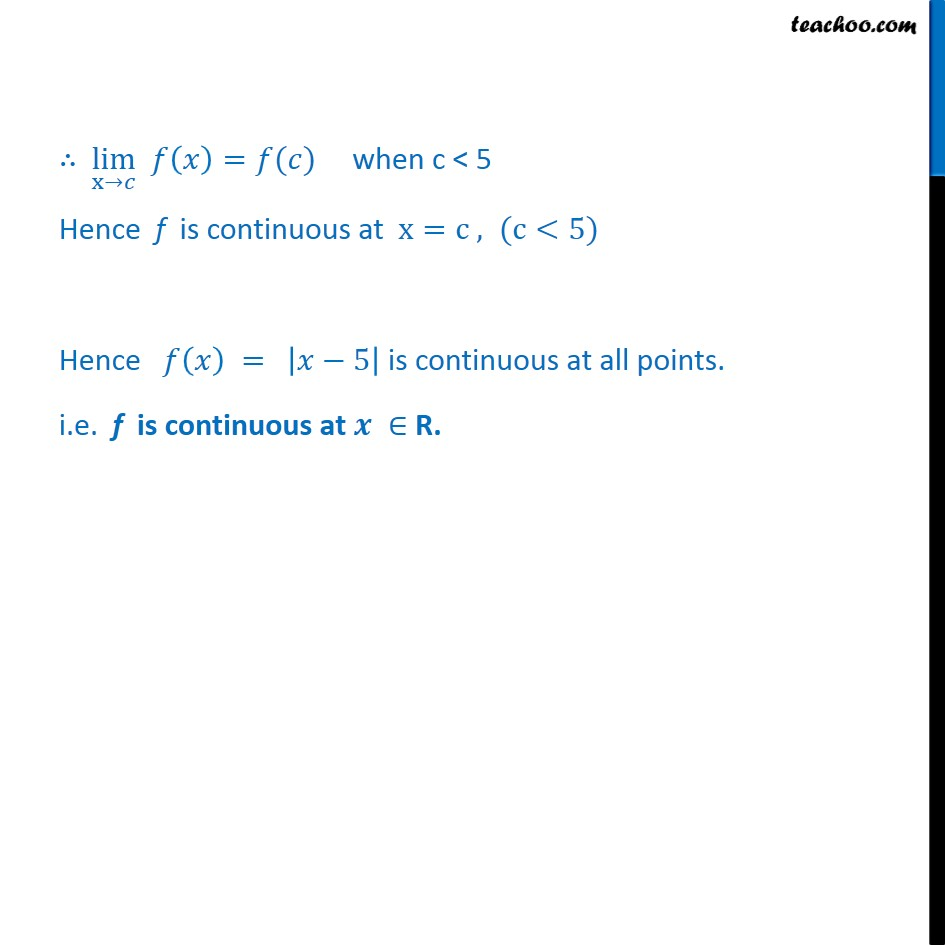1. Chapter 5 Class 12 Continuity and Differentiability
2. Serial order wise
3. Ex 5.1

Transcript

Ex 5.1, 3 Examine the following functions for continuity. (a) f (x) = x – 5 f (x) = x – 5 Since x – 5 is a polynomial. f is defined for every real number c. Given function is continuous at x = c , where c is a real number if, ﷐lim﷮x→𝑐﷯ 𝑓(𝑥) = 𝑓(𝑐) Since, ﷐lim﷮x→𝑐﷯ = f (c) So, f is continuous for x = c, where c is a real number ∴ f is continuous for all real numbers ⇒ f is continuous at each x ∈ R Ex 5.1, 3 Examine the following functions for continuity. (b) f (x) = ﷐1﷮𝑥 − 5﷯ , x ≠ 5 If x = 5 f (x) = ﷐1﷮5 − 5﷯ = ﷐1﷮0﷯ = 𕔴 Hence at x = 5, f (x) is not defined. Given function is continuous at x = c , where c is a real number except 5 if ﷐lim﷮x→𝑐﷯ 𝑓﷐𝑥﷯ = 𝑓﷐𝑐﷯ Hence for all Real number except 5, ﷐lim﷮x→𝑐﷯ 𝑓﷐𝑥﷯ = 𝑓﷐𝑐﷯ f is continuous for all real numbers except 5 ∴ f is continuous at each 𝐱 ∈ R − ﷐𝟓﷯ Ex 5.1, 3 Examine the following functions for continuity. (c) f (x) = ﷐﷐𝑥﷮2 ﷯− 25 ﷮𝑥 + 5﷯, x ≠ –5 f (x) = ﷐﷐𝑥﷮2 ﷯− 25 ﷮𝑥 + 5﷯ x ≠ –5 Putting x = –5 f (−5) = ﷐﷐(−5)﷮2 ﷯− 25 ﷮−5 + 5﷯ = ﷐25− 25 ﷮−5 + 5﷯ = ﷐0﷮0﷯ = undefined Hence At 𝑥 =−5 , 𝑓﷐𝑥﷯ is not defined. Given function is continuous at x = c , where c is a real number except −5 if ﷐lim﷮x→𝑐﷯ 𝑓(𝑥) = 𝑓(𝑐) Since, ﷐lim﷮x→𝑐﷯ 𝑓﷐𝑥﷯ = f (c) f is continuous for all real numbers except −5 ∴ f is continuous at each 𝐱 ∈ R − ﷐−𝟓﷯ Ex 5.1, 3 Examine the following functions for continuity. (d) f (x) = |x – 5| f (x) = ﷐𝑥−5﷯ Case 1 Checking continuity At 𝑥 = 5 f is continuous at 𝑥 = 5 if L.H.L = R.H.L = 𝑓﷐5﷯ if ﷐lim﷮x→﷐5﷮−﷯﷯ 𝑓﷐𝑥﷯=﷐lim﷮x→﷐5﷮+﷯﷯ 𝑓(𝑥)= 𝑓(5) & 𝑓(5) = ﷐𝑥−5﷯ = ﷐5−5﷯ = ﷐0﷯ = 0 Hence, L.H.L = R.H.L = 𝑓﷐5﷯ ∴ f is continuous at x=5 Case 2 Checking continuity At 𝑥 =𝑐 𝑥 =𝑐 where c > 5 𝑓﷐𝑥﷯= ﷐𝑥−5﷯ 𝑓﷐𝑥﷯= x − 5 f is continuous at x=c (c>5) if if ﷐lim﷮x→𝑐﷯ 𝑓﷐𝑥﷯=𝑓(𝑐) Since ﷐lim﷮x→𝑐﷯ 𝑓﷐𝑥﷯=𝑓(𝑐) Hence f is continuous at x=c , c>5 Case 3 Checking continuity At 𝑥 =𝑐 (c < 5) Consider x = c, c < 5 𝑓﷐𝑥﷯ = ﷐𝑥−5﷯ 𝑓﷐𝑥﷯ = −﷐𝑥−5﷯ f is continuous at x=c , c<5 if ﷐lim﷮x→𝑐﷯ 𝑓﷐𝑥﷯=𝑓(𝑐) ∴ ﷐lim﷮x→𝑐﷯ 𝑓﷐𝑥﷯=𝑓(𝑐) when c < 5 Hence f is continuous at x=c , (c<5) Hence 𝑓﷐𝑥﷯ = ﷐𝑥−5﷯ is continuous at all points. i.e. f is continuous at 𝒙 ∈ R.

Ex 5.1